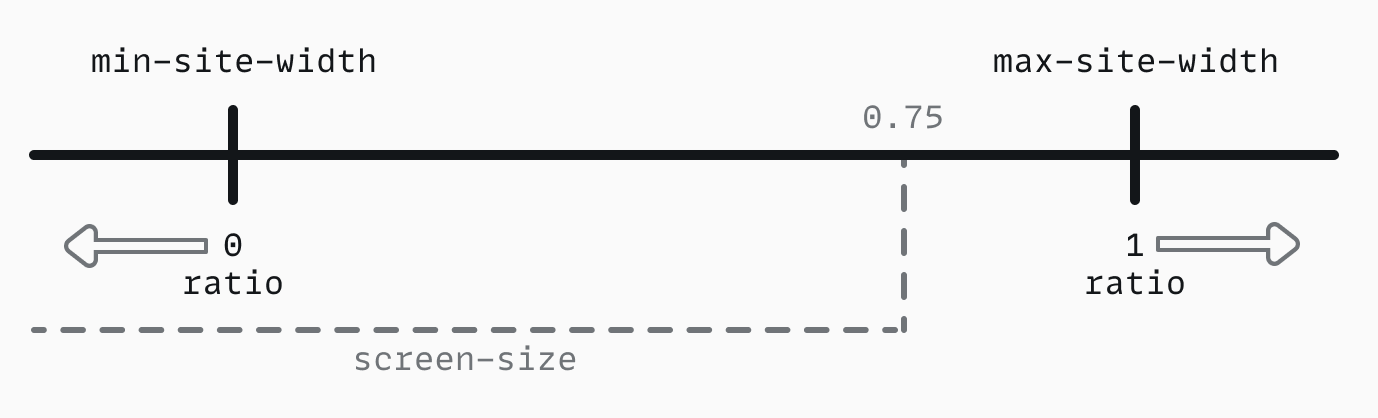# Fluid interfaces using CSS

Responsiveness should be a core feature of any website. Screen-sizes are both growing and shrinking. This makes that responsiveness now goes beyond composition and layout. We need to adjust the sizes of text and elements and the surrounding spacing. Most achieve this by adding media-queries. But with various pages, elements, and breakpoints, adding media-queries become unmaintainable.

When refactoring crinkles.io I wanted to remove the dependency on media-queries. But, I wanted sizing and spacing adjust based on the screen size. I needed fluidity on my website. By utilizing CSS variables and the CSS `calc` function, I could achieve this. Check out how!

## Fluid sizing and spacing

I was using media-queries for spacing values (e.g. `padding` and `margin`) and font-sizes. On big screens I want larger font-sizes and spacing compared to mobile. The goal is to improve usability and reduce wasted space. But with media-queries, I had to go over all different breakpoints and determine how I wanted to change the spacing and font-sizes. Introducing, fluid interfaces.

With fluid interfaces, you use linear interpolation to scale attributes when your website increases in size automatically (e.g. between `16px` and `20px`). To achieve this, we need to determine a ratio (a value between 0 and 1) which we can use to scale values based on the website size.To determine the ratio, you first need to determine the min. and max. width your site has. Some sites take up all the available horizontal space, but many have a max. width. With a min. and max. determined, we can calculate the ratio with the equation below. With this ratio, we can interpolate a size between two values.

``````         min(100vw, max) - min
ratio = -----------------------
max - min``````

With this ratio, we can scale any size (e.g. font-sizes or spacing) based on how big the horizontal size of our website is.

``size = min + (max - min) × ratio``

## Calculating the ratio with `calc`

To implement this concept, you need CSS variables and the `calc` function. Although it seems easy enough, the implementation comes with some quirks. But first, let's determine our base values. In this implementation all sizes are unitless or in `rem` values. Based on this value, we can set our initial variables.

```css```/* based on 16px */
:root {
--unit: 1rem;
--min: 20;
--max: 75;
}``````

With the base values known, we can start calculating the ratio. Here we find the quirks we need to handle. We need to use the `min` CSS function to calculate the `--area` value we can determine the ratio with. But, this function always needs values with units for comparison. Thus we multiply our `--max` with the `--unit`.

The `--area` variable is the left-hand side of the described ratio equation. Now we can calculate the `--ratio`. Because `--area` already has a unit of `rem`, `--ratio` will be a value between `0rem` and `1rem`.

```css```/* ratio calculation */
:root {
--screen: calc(min(100vw, var(--max) * 1rem));
--area: calc(var(--screen) - var(--min) * 1rem);
--ratio: calc(var(--area) / (var(--max) - var(--min)));
}``````

With a ratio based on the actual screen size between `0rem` and `1rem`, we can start scaling elements on our website. For this, we used the described scale equation. Let's look at the example below. This example calculates a build font-size for an article.

```css```/* example for a fluid article font-size */
:root {
--min-size: 1.125rem;
--max-size: 1.375rem;
--text: calc(var(--max-size) - var(--min-size));
--size: calc(var(--min-size) + var(--text) * var(--ratio));
}``````

On small screens, the font-size will be `1.125rem` and on big screens `1.375rem`. With a base font-size of `16px`, we can now calculate the exact font-sizes for different screen-sizes. A screen-size of `784px` will have an article font-size of `20.109px`. But font-sizes is one of many places where we can apply fluidity.

• Font-sizes for paragraphs and headers.
• Various spacing values used between and around elements.
• Sizing of the site's logo and icons.

All can have their own set of calculations for fluidity. These can be separate design tokens, or you can scale from the parent element (e.g. using `em` instead of `rem`).

## Conclusion

When refactoring crinkles.io I wanted to reduce the number of media-queries, but maintain responsiveness. I wanted to reduce the CSS footprint of the website. But above all, I wanted to make my CSS more maintainable. Adding fluidity for font-sizes, element sizes, and spacing contributed to achieving this goal. Want to see this fluidity in action? Just open this article on your laptop or PC, and slowly resize the browser window.

← Next

Previous →